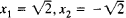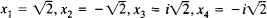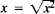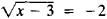# equation

(redirected from Axioms of equation)
Also found in: Dictionary, Thesaurus, Medical, Financial.

## equation

equation, in mathematics, a statement, usually written in symbols, that states the equality of two quantities or algebraic expressions, e.g., x+3=5. The quantity x+3, to the left of the equals sign (=), is called the left-hand, or first, member of the equation, that to the right (5) the right-hand, or second, member. A numerical equation is one containing only numbers, e.g., 2+3=5. A literal equation is one that, like the first example, contains some letters (representing unknowns or variables). An identical equation is a literal equation that is true for every value of the variable, e.g., the equation (x+1)2=x2+2x+1. A conditional equation (usually referred to simply as an equation) is a literal equation that is not true for all values of the variable, e.g., only the value 2 for x makes true the equation x+3=5. To solve an equation is to find the value or values of the variable that satisfy it. Polynomial equations, containing more than one term, are classified according to the highest degree of the variable they contain. Thus the first example is a first degree (also called linear) equation. The equation ax2+bx+c=0 is a second degree, or quadratic, equation in the unknown x if the letters a, b, and c are assumed to represent constants. In algebra, methods are evolved for solving various types of equations. To be valid the solution must satisfy the equation. Whether it does can be ascertained by substituting the supposed solution for the variable in the equation. The simultaneous solution of two or more equations is a set of values of the variables that satisfies each of the equations. In order that a solution may exist, the number of equations (i.e., conditions) must generally be no greater than the number of variables. In chemistry an equation (see chemical equation) is used to represent a reaction.
The following article is from The Great Soviet Encyclopedia (1979). It might be outdated or ideologically biased.

## Equation

in mathematics, the analytical form of the problem of finding those inputs for which two given functions have equal outputs. The functional inputs are usually called unknowns, and the unknowns for which the functional outputs are equal are called solutions or roots. We say that the solutions satisfy the given equation. For example 3x – 6 = 0 is an equation in one unknown with solution x = 2, and x2 + y2 = 25 is an equation in two unknowns, one of whose solutions is x = 3, y = 4. The totality of solutions of an equation depends on the set M of values that may be assigned to the unknowns. An equation may have no solution in M, or it may have some, or even infinitely many, solutions in M. For example, the equation x4 – 4 = 0 has no rational solutions, two real solutions, and four complex solutions. The equation sin x = 0 has infinitely many real solutions xk = kπ (k = 0, ±1, ±2, . . .). If an equation is satisfied by every number in M, then we say that it is an identity over M. For example,is an identity over the non-negative reals but not over the reals.

When we are looking for values of the unknowns that satisfy a set of equations, then we call this set a system of equations, and the numbers in question, solutions of the system. For example, the equations x + 2y = 5, 2x + y – z = 1 are a system or two equations in three unknowns. One solution of this system is x = 1, y = 2, z = 3.

Two systems (two equations) are said to be equivalent if every solution of one system (one equation) is a solution of the other system (the other equation) and conversely. Here we require that both systems (equations) be considered over the same input domain. For example, the equations x – 4 = 0 and 2x – 8 = 0 are equivalent, since their common solution is x = 4. Every system of equations is equivalent to a system of the form fk (x1, x2, ..., xn) = 0, k = 1, 2, . . . . When finding the solutions of an equation, we usually replace it with an equivalent equation. In some cases it is necessary to replace the given equation with an equation that has more solutions than the given equation. A solution of the new equation that is not a solution of the given equation is called extraneous (seeEXTRANEOUS ROOT). For example, by squaring the equation, we obtain the equation x – 3 = 4 whose solution x = 7 is extraneous for the original equation. Thus, if in the process of solving an equation we resort to steps—such as squaring—that may introduce extraneous roots, then the solutions of the transformed equation must be verified by substitution in the original equation.

We know most about equations for which the functions fk are polynomials in variables x1, x2,..., xn, that is, algebraic equations. For example, an algebraic equation in one unknown has the form

(*) a0xn + a1xn–1 + ... + an = 0 (a0 ≠ 0)

The number n is called the degree of the equation. Solutions of equations of degree 1 and 2 were known in antiquity. The problem of solving an algebraic equation of degree n was one of the most important problems of the 16th and 17th centuries. During that time, mathematicians developed methods for solving equations of degrees 3 and 4 and obtained formulas for the roots of such equations (seeALGEBRA and CARDANO’S FORMULA). No such formulas exist for equations of degree n ≥ 5, since, in general, such equations cannot be solved in radicals. This fact was proved by N. Abel in 1824. About 1830, consideration of the problem of the solvability of algebraic equations in radicals led E. Galois to a general theory of algebraic equations.

Every algebraic equation has at least one real or complex solution. This assertion is the fundamental theorem of algebra, first proved by K. Gauss in 1799. If α is a solution of the equation (*), then the polynomial a0xn + a1xn–1 + ... + an is divisible by x – α. If it is divisible by (x – α)k but not by (x – α)K + 1, then we say that a is a root of multiplicity k. Counting multiplicities, the number of solutions of the equation (*) is n.

If the function f(x) is transcendental, then the equation f(x) = 0 is called a transcendental equation (as an example, seeKEPLER’S EQUATION). Depending on the form of f(x), we distinguish trigonometric, logarithmic, and exponential equations. We also consider equations with irrationalities, that is, expressions under radicals. The practical solution of equations involves various approximation methods.

The simplest system of equations is a system of linear equations in which the fk are polynomials of degree 1 in x1, x2, ..., xn (seeLINEAR EQUATION).

In general, the solution of a system of (not necessarily linear) equations reduces to the solution of a single equation by means of the method of elimination of unknowns (see alsoRESULTANT).

In analytic geometry we interpret an equation in two unknowns as a plane curve consisting of all points whose coordinates satisfy the given equation. We interpret an equation in three unknowns as a surface in 3-space. In this interpretation, the solution of a system of equations reduces to the problem of finding the points of intersection of curves, surfaces, and so on. Equations in more than three unknowns must be interpreted as sets of points in n-dimensional spaces.

In number theory we consider indeterminate equations, that is, equations in several unknowns for which we wish to find solutions that are integers or rational numbers (seeDIOPHANTINE EQUATIONS). For example, the integer solutions of the equation x2 + y2 = z2 are of the form x = m2n2; y = 2mn; z = m2 + n2, where m and n are integers.

Let F and Φ be mappings of a set A into a set B. From the most general point of view, an equation is the statement of the problem of finding elements a in A such that F(a) = Φ(a). If A and B are sets of numbers, then we obtain an equation of the type considered above. If A and B are sets in multidimensional spaces, then we obtain systems of equations. Finally, if A and B are sets of functions, then, depending on the nature of the mappings, we can also obtain differential, integral and other forms of equations (seeDIFFERENTIAL EQUATIONS and INTEGRAL EQUATIONS). In addition to the problem of finding solutions of an equation, we consider problems of existence and uniqueness of a solution as well as the problem of the continuous dependence of a solution on various data.

The term “equation” is also used in other natural sciences in a sense different from’ that above. Relevant examples are the time equation in astronomy, the equation of state in physics, chemical equations, Maxwell’s equations in electrodynamics, and the Boltzmann kinetic equation in the theory of gases.

## equation

[i′kwā·zhən]
(chemistry)
A symbolic expression that represents in an abbreviated form the laboratory observations of a chemical change; an equation (such as 2H2+ O2→ 2H2O) indicates what reactants are consumed (H2 and O2) and what products are formed (H2O), the correct formula of each reactant and product, and satisfies the law of conservation of atoms in that the symbols for the number of atoms reacting equals the number of atoms in the products.
(mathematics)
A statement that each of two expressions is equal to the other.
McGraw-Hill Dictionary of Scientific & Technical Terms, 6E, Copyright © 2003 by The McGraw-Hill Companies, Inc.

## equation

1. a mathematical statement that two expressions are equal: it is either an identity in which the variables can assume any value, or a conditional equation in which the variables have only certain values (roots)
Collins Discovery Encyclopedia, 1st edition © HarperCollins Publishers 2005

## equation

An arithmetic expression that equates one set of conditions to another; for example, A = B + C. In a programming language, assignment statements take the form of an equation. The above example assigns the sum of B and C to the variable A. See formula editor.
Copyright © 1981-2019 by The Computer Language Company Inc. All Rights reserved. THIS DEFINITION IS FOR PERSONAL USE ONLY. All other reproduction is strictly prohibited without permission from the publisher.
Site: Follow: Share:
Open / Close